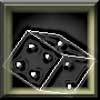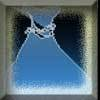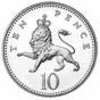#### You may also like### Gambling at Monte Carlo

A man went to Monte Carlo to try and make his fortune. Is his strategy a winning one?### Marbles and Bags

Two bags contain different numbers of red and blue marbles. A marble is removed from one of the bags. The marble is blue. What is the probability that it was removed from bag A?### Coin Tossing Games

You and I play a game involving successive throws of a fair coin. Suppose I pick HH and you pick TH. The coin is thrown repeatedly until we see either two heads in a row (I win) or a tail followed by a head (you win). What is the probability that you win?

# Jewellery Boxes

##### Age 14 to 16 ShortChallenge Level

Suppose that initially the jewels in $P$ are worth $(a + b + 5000)$,
and those in $Q$ are worth $(c + d + e)$.

The average value in $P$ at the start is $\frac {a + b + 5000}3$

After the jewel has been moved it is $\frac{a + b}2$.
Therefore:
\begin{equation}
\frac {a + b + 5000}3 = \frac{a + b }{2} - 1000
\end{equation}
Multiplying by $6$ and collecting like terms gives $a + b = 16000$

The average value in $Q$ at the start is $\frac {c + d + e}3$

After the jewel has been moved, the average value is $\frac{c + d + e + 5000}4$
Therefore:
\begin{equation}
\frac {c + d + e}3 = \frac{c + d + e + 5000}{4} - 1000
\end{equation}
Multiplying by $12$ and collecting like terms gives $c + d + e = 3000$.

Therefore the total value is
$$a + b + 5000 + c + d + e = 16000 + 5000 + 3000 = 24000$$

This problem is taken from the UKMT Mathematical Challenges.
You can find more short problems, arranged by curriculum topic, in our short problems collection.